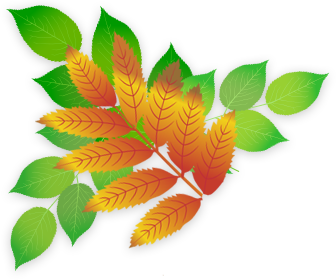## Developing Numeracy in Year 5

In Year 5 children work with numbers to at least 1,000,000. They will multiply and divide numbers up to 4 digits using formal written methods. They are taught to use negative numbers in context and understand prime numbers and prime factors. They will work confidently with decimal numbers and understand how fractions relate to decimal numbers and percentages. They will be taught to add and subtract fractions and recognise which fractions are equivalent. Children will be expected draw and measure angles to 360 degrees and will learn how to draw and reflect shapes. They will be taught to solve problems involving money and present information in a graphs, tables and charts.

These are the objectives that children will be taught in Numeracy during Year 5.

Year 5: Number and Place Value

• find 1000 more or less than a given number
• count backwards through zero to include negative numbers
• recognise the place value of each digit in a four-digit number (thousands, hundreds, tens, and ones)
• order and compare numbers beyond 1000
• identify, represent and estimate numbers using different representations
• round any number to the nearest 10, 100 or 1000
• solve number and practical problems that involve all of the above and with increasingly large positive numbers

• add and subtract whole numbers with more than 4 digits, including using formal written methods (columnar addition and subtraction)
• add and subtract numbers mentally with increasingly large numbers
• use rounding to check answers to calculations and determine, in the context of a problem, levels of accuracy
• solve addition and subtraction multi-step problems in contexts, deciding which operations and methods to use and why.

Year 5: Multiplication and Division

• identify multiples and factors, including finding all factor pairs of a number, and common factors of 2 numbers
• know and use the vocabulary of prime numbers, prime factors and composite (non-prime) numbers
• establish whether a number up to 100 is prime and recall prime numbers up to 19
• multiply numbers up to 4 digits by a one- or two-digit number using a formal written method, including long multiplication for two-digit numbers
• multiply and divide numbers mentally, drawing upon known facts
• divide numbers up to 4 digits by a one-digit number using the formal written method of short division and interpret remainders appropriately for the context
• multiply and divide whole numbers and those involving decimals by 10, 100 and 1,000
• recognise and use square numbers and cube numbers, and the notation for squared (²) and cubed (³)
• solve problems involving multiplication and division, including using their knowledge of factors and multiples, squares and cubes
• solve problems involving addition, subtraction, multiplication and division and a combination of these, including understanding the meaning of the equals sign
• solve problems involving multiplication and division, including scaling by simple fractions and problems involving simple rates

Year 5: Fractions (including decimals and percentages)

• compare and order fractions whose denominators are all multiples of the same number
• identify, name and write equivalent fractions of a given fraction, represented visually,including tenths and hundredths
• recognise mixed numbers and improper fractions and convert from one form to the other and write mathematical statements >1 as a mixed number [for example,2⁄5+4⁄5=6⁄5= 11⁄5]
• add and subtract fractions with the same denominator and denominators that are multiples of the same number
• multiply proper fractions and mixed numbers by whole numbers, supported by materials and diagrams
• read and write decimal numbers as fractions [for example, 0.71 =71⁄100]
• recognise and use thousandths and relate them to tenths, hundredths and decimal equivalents
• round decimals with two decimal places to the nearest whole number and to one decimal place
• read, write, order and compare numbers with up to three decimal places
• solve problems involving number up to three decimal places
• recognise the per cent symbol (%) and understand that per cent relates to ‘number of parts per hundred’, and write percentages as a fraction with denominator 100, and as a decimal
• solve problems which require knowing percentage and decimal equivalents of 1⁄2, 1⁄4,1⁄5, 2⁄5 and those fractions with a denominator of a multiple of 10 or 25.

Year 5: Measurement

• convert between different units of metric measure (for example, kilometre and metre; centimetre and metre; centimetre and millimetre; gram and kilogram; litre and millilitre)
• understand and use approximate equivalences between metric units and common imperial units such as inches, pounds and pints
• measure and calculate the perimeter of composite rectilinear shapes in centimetres and metres
• calculate and compare the area of rectangles (including squares), and including using standard units, square centimetres (cm2) and square metres (m2) and estimate the area of irregular shapes
• estimate volume [for example, using 1 cm3 blocks to build cuboids (including cubes)] and capacity [for example, using water]
• solve problems involving converting between units of time
• use all four operations to solve problems involving measure [for example, length, mass, volume, money] using decimal notation, including scaling.

Year 5: Geometry - properties of shapes

• identify 3-D shapes, including cubes and other cuboids, from 2-D representations
• know angles are measured in degrees: estimate and compare acute, obtuse and reflex angles
• draw given angles, and measure them in degrees (°)
• identify:
• use the properties of rectangles to deduce related facts and find missing lengths and angles
• distinguish between regular and irregular polygons based on reasoning about equal sides and angles.

Year 5: Geometry - position and direction

• identify, describe and represent the position of a shape following a reflection or translation, using the appropriate language, and know that the shape has not changed.

Year 5: Statistics

• solve comparison, sum and difference problems using information presented in a line graph
• complete, read and interpret information in tables, including timetables.